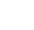Your current browser version is too low, in order to bring you a better browsing effect, please use a higher version browser

You Are Here: home-Blog

# Medium Frequency Induction Furnace Power Calculation

Medium frequency induction furnaces are widely used in various industries for melting and heating purposes. The calculation of power required for these furnaces is crucial in determining their efficiency and performance. In this article, we will discuss the power calculation process for medium frequency induction furnaces and its significance in optimizing their operations.

Power Calculation Method:
The power calculation for medium frequency induction furnaces involves several factors, including the material being melted, the desired melting rate, and the furnace’s efficiency. The formula commonly used for power calculation is as follows:

Power (in kilowatts) = (Melt Rate × Heat of Fusion) / Furnace Efficiency

1. Melt Rate:
The melt rate is the amount of material melted per unit of time. It can be calculated by dividing the weight of the material by the melting time. For example, if 1000 kilograms of material is melted in 2 hours, the melt rate would be 500 kilograms per hour.

2. Heat of Fusion:
The heat of fusion is the amount of energy required to change a substance from a solid to a liquid state at its melting point. It is expressed in joules per kilogram (J/kg) or calories per gram (cal/g). The heat of fusion varies for different materials and can be obtained from reference tables or literature.

3. Furnace Efficiency:
Furnace efficiency refers to the percentage of energy that is effectively utilized in the melting process. It takes into account losses due to factors such as radiation, conduction, and convection. Furnace efficiency can be influenced by the design, insulation, and operating conditions of the furnace.

Significance of Power Calculation:
Accurate power calculation is essential for optimizing the operations of medium frequency induction furnaces. It helps in determining the appropriate power capacity required for a specific melting task, avoiding overloading or underutilization of the furnace. By calculating the power accurately, the furnace can operate at its maximum efficiency, resulting in reduced energy consumption and improved productivity.

Moreover, power calculation aids in estimating the operating costs associated with the furnace. It enables businesses to evaluate the financial feasibility of using medium frequency induction furnaces for their specific melting requirements. Additionally, power calculation can assist in identifying potential areas for improvement and optimization in furnace operations.

Conclusion:
Medium frequency induction furnace power calculation plays a vital role in determining the efficiency, productivity, and operating costs of these furnaces. By accurately calculating the power required, businesses can optimize their furnace operations, reduce energy consumption, and enhance productivity. It is important to consider the melt rate, heat of fusion, and furnace efficiency while performing the power calculation.